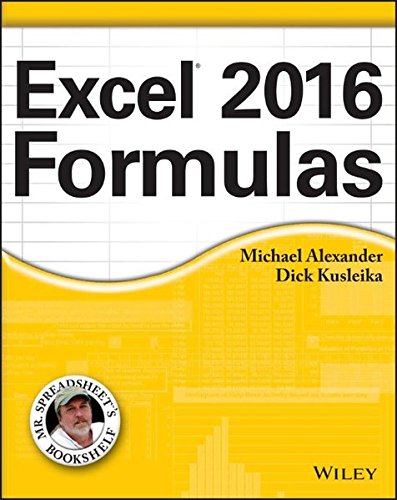# Excel 2016 Formulas

• Length: 816 pages
• Edition: 1
• Language: English
• Publisher:
• Publication Date: 2016-01-19
• ISBN-10: 1119067863
• ISBN-13: 9781119067863
4.2

## Book Description

Leverage the full power of Excel formulas

"Excel 2016 Formulas" is fully updated to cover all of the tips, tricks, and techniques you need to maximize the power of Excel 2016 through the use of formulas. This comprehensive book explains how to create financial formulas, release the power of array formulas, develop custom worksheet functions with VBA, debug formulas, and much more. Whether you're a beginner, a power user, or somewhere in between this is your essential go-to for the latest on Excel formulas.

When conducting simple math or building highly complicated spreadsheets that require formulas up to the task, leveraging the right formula can heighten the accuracy and efficiency of your work, and can improve the speed with which you compile and analyze data. Understanding which formulas to use and knowing how to create a formula when you need to are essential. Access tips, tricks, and techniques that have been fully updated to reflect the latest capabilities of Microsoft Excel Create and use formulas that have the power to transform your Excel experience Leverage supplemental material online, including sample files, templates, and worksheets from the book

Part I: Understanding Formula Basics
Chapter 1: The Excel User Interface in a Nutshell
Chapter 2: Basic Facts About Formulas
Chapter 3: Working with Names

Part II: Leveraging Excel Functions

Chapter 4: Introducing Worksheet Functions
Chapter 5: Manipulating Text
Chapter 6: Working with Dates and Times
Chapter 7: Counting and Summing Techniques
Chapter 8: Using Lookup Functions
Chapter 9: Working with Tables and Lists
Chapter 10: Miscellaneous Calculations

Part III: Financial Formulas
Chapter 11: Borrowing and Investing Formulas
Chapter 12: Discounting and Depreciation Formulas
Chapter 13: Financial Schedules

Part IV: Array Formulas
Chapter 14: Introducing Arrays
Chapter 15: Performing Magic with Array Formulas

Part V: Miscellaneous Formula Techniques
Chapter 16: Importing and Cleaning Data
Chapter 17: Charting Techniques
Chapter 18: Pivot Tables
Chapter 19: Conditional Formatting
Chapter 20: Using Data Validation
Chapter 21: Creating Megaformulas
Chapter 22: Tools and Methods for Debugging Formulas

Part VI: Developing Custom Worksheet Functions
Chapter 23: Introducing VBA
Chapter 24: VBA Programming Concepts
Chapter 25: Function Procedure Basics
Chapter 26: VBA Custom Function Examples

Part VII: Appendixes
Appendix A: Excel Function Reference
Appendix B: Using Custom Number Formats